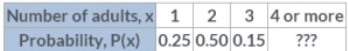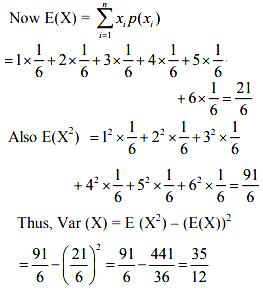# Test: Mean And Variance Of A Random Variable

## 10 Questions MCQ Test Mathematics (Maths) Class 12 | Test: Mean And Variance Of A Random Variable

Description
This mock test of Test: Mean And Variance Of A Random Variable for JEE helps you for every JEE entrance exam. This contains 10 Multiple Choice Questions for JEE Test: Mean And Variance Of A Random Variable (mcq) to study with solutions a complete question bank. The solved questions answers in this Test: Mean And Variance Of A Random Variable quiz give you a good mix of easy questions and tough questions. JEE students definitely take this Test: Mean And Variance Of A Random Variable exercise for a better result in the exam. You can find other Test: Mean And Variance Of A Random Variable extra questions, long questions & short questions for JEE on EduRev as well by searching above.
QUESTION: 1

### In a series of 2 n observations, half of them equal ‘a’ and remaining half equal – a. If the standard deviation of the observations is 2, then | a | equals ……​

Solution:

Here, mean = [na+n(−a)]/2n=0
Hence variance = [na2+n(−a)2]2n − (mean)2
⇒ 2 = a2 − (0)2
⇒ a= 2

QUESTION: 2

Solution:
QUESTION: 3

### The number of adults living in homes on a randomly selected city block is described by the following probability distribution.What is the probability that 4 or more adults reside at a randomly selected home?​

Solution:

The sum of all the probabilities is equal to 1.
Therefore, the probability that four or more adults reside in a home = 1 - (0.25 + 0.50 + 0.15)
= 0.10.

QUESTION: 4

Two dice are thrown simultaneously. If X denotes the number of sixes, then the expectation of X is:​

Solution:

Here, X represents the number of sixes obtained when two dice are thrown simultaneously. Therefore, X can take the value of 0, 1, or 2.
∴ P(X=0)=P(not getting six on any of the dice) = 25/36
P(X=1)=P(sixo n first die and no six on second die) + P(no six on firstdie and six on second die)
=2(1/6 × 5/6)=10/36
P(X=2)=P(sixonboththedice)= 1/36
Therefore, the required probability distribution is as follows.
Then, expectation of X=E(X)=∑Xi
​P(Xi)= 0(25/36)+1(10/36)+2(1/36)
= 1/3

QUESTION: 5

Let X be a random variable whose possible values x1, x2, x3, …, xn occur with probabilities p1, p2, p3,…, pn, respectively. Also, μ be the mean of X. The variance of X, denoted by Var (X) is defined as

Solution:
QUESTION: 6

The variance of the number obtained on a throw of an unbiased dice is:​

Solution:

The sample space of the experiment is S = {1, 2, 3, 4, 5, 6}.
Let X denote the number obtained on the throw.
Then X is a random variable which can take values X = 1, 2, 3, 4, 5, or 6.  Also P(1) = P(2) = P(3) = P(4) = P(5) = ⅙QUESTION: 7

The mean number of tails in three tosses of a fair coin is:​

Solution:

Let X denotes the number of tails, then probability distribution is
X         0      1      2     3
P(X)    1/8  3/8  3/8  1/8
Mean (xipi) = 0(⅛) + 1(⅜) + 2(⅜) + 3(⅛)
= 3/2

QUESTION: 8

A class has 10 students whose ages are 15, 14, 16, 17, 19, 20, 16, 18, 20, and 20 years. One student is selected in such a manner that each has the same chance of being chosen and the age X of the selected student is recorded. The standard deviation of X is:​

Solution:
QUESTION: 9

Let X be a random variable whose possible values x1, x2, x3, …, xn occur with probabilities p1, p2, p3,…, pn, respectively. Also, E(X) is the expectation of X, then ​E(X2) - [E(X)]2 is known as

Solution:
QUESTION: 10

In a meeting, 60% of the members favour and 40% oppose a certain proposal. A member is selected at random and we take X = 0 if he opposed, and X = 1 if he is in favour. Find E(X) and Var(X).​

Solution: